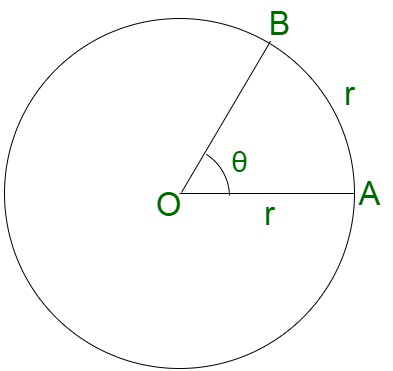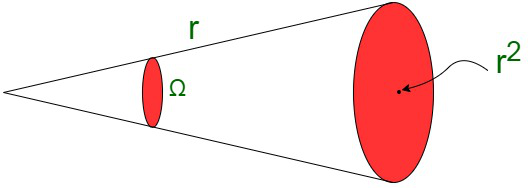GeeksforGeeks App
Open AppBrowser
Continue

## Related Articles

• CBSE Class 11 Physics Notes

Measurement forms the fundamental principle to various other branches of science, that is, construction and engineering services. Measurement is defined as the action of associating numerical with their possible physical quantities and phenomena. Measurements find a role in everyday activities to a large extent. Therefore, it is necessary to study and explore the associated elements along with their theoretical foundations, conditions as well as limitations. It defines the units to be chosen for the measurement of various commodities. It also caters to the comparison of plausible units with the ones already existing of a similar kind.

Measurement defined the new standards as well as form transductions for the quantities which do not have any possible access for direct comparison. These physical quantities can be converted into analogous measurement signals.

Measurements may be made by unaided human senses, generally termed as estimates. It can also be estimated by the use of instruments, which may range in complexity from simple rules for measuring lengths to highly complex analogous systems to handle and design the commodities beyond the capabilities of the senses. Thus, the measurements may range from buying some quantity of milk (in L) or to the highly complex mechanisms, such as radio waves from a distant star or the nuclear bomb radiations. Therefore, we can consider that a measurement, always involves a transfer of energy or interaction between the object and the observer or observing instrument.Measurement of Height of a person

Unit

The unit of a specified physical quantity can be considered as an arbitrarily chosen standard that can be used to estimate the quantities belonging to similar measurements. The units are well accepted and recognized by the people and well within all guidelines.

A physical quantity is measured in terms of the chosen standards of measurement.

The chosen standard is recognized as the unit of that corresponding physical quantity. A standard unit, in short, is a definite amount of a physical quantity. These standard units can be quickly reproduced to create a wide variety of units and are internationally accepted and accessible.

The measurement of any physical quantity is based on a formula, nu,

where, n = numerical value of the measure of the quantity,

u = unit of the quantity.

Standard

The actual physical embodiment of the unit of a physical quantity is termed as a standard of that physical quantity. The standard is expressed in terms of the numerical value (n) and the unit (μ).

Measurement of physical quantity = Numerical value × Unit

For example: Length of a rod = 12 m. Here 12 is its numerical segment and m (meter) is the unit.

Fundamental Units

Fundamental units are elementary in nature, that is, they can be expressed independently without any dependence on any other physical quantity. This implies that it is not possible to resolve it further in terms of any other physical quantity. It is also termed as a basic physical quantity. Fundamental quantities have their own values and units.

Supplementary Fundamental Units

There are two other supplementary fundamental units, namely Radian and steradian are two supplementary which measures plane angle and solid angle respectively.

One radian is equivalent to an angle subtended at the center of a circle by an arc of length equal to the radius of the circle. It is the unit represented for the plane angle.One steradian is equivalent to the solid angle subtended at the center of a sphere by its surface. Its area is equivalent to the square of the radius of the sphere.It is the unit represented for the solid angle. Solid angle in steradian,Properties of Fundamental Units

Any standard unit should have the following two properties:

• Invariability
The standard unit must be invariable. Thus, defining distance between the tip of the middle finger and the elbow as a unit of length is not invariable.
• Availability
The standard unit should be easily made available for comparing with other quantities.

The seven fundamental units of S.I. have been defined as under.

• Meter (m)
Defined as 1650763.73 times the wavelength, in vacuum of the orange light emitted in transition from 2p10­  to 5d5.
• Kilogram (kg)
Defined as the mass of a platinum-iridium cylinder kept at Serves.
• Second (s)
Time taken by 9192631770 cycles of the radiation from the hyperfine transition in cesium – 133 when unperturbed by external fields.
• Ampere (A)
The constant current which, if maintained in each of two infinitely long, straight, parallel wires of negligible cross-section placed 1 m apart, in vacuum, produces between the wires a force of 2×10-7 newton per meter length of the wires.
• Kelvin (K)
Temperature is measured with absolute zero as the zero and the triple point of water as the upper fixed point on the thermodynamic scale. The interval is divided into 273.15 divisions and each division is considered to be unit temperature.
• Candela (cd)
The luminous intensity in the perpendicular direction of a surface ofsquare meter of a full radiator at the temperature of freezing platinum under a pressure of 101325 newtons per square meter.
• Mole (mol)
The mole is the amount of any substance which contains as many elementary entities as there are atoms in 0.012 kg of the carbon isotopeC.

Derived units

The derived units are in usage for the commodities where the units are obtained from a combination of fundamental units. Derived units are sometimes assigned names. For instance, the S.I unit of force is kg ms-2 , termed as Newton (N). The unit of power is kg m2 s-3 , termed as watt (W).

Steps to find Derived Units

• Fetch the formula for the quantity whose unit is to be derived.
• Substitute units of all the involved quantities. The chosen units should all belong to one system on units in their fundamental or standard form.
• Simplify for the derived unit of the quantity to compute its final unit.

Example: Compute the unit of velocity.

Since, we know velocity is a derived quantity, obtained from distance and time(fundamental quantities).

Mathematically ,

velocity =  displacement/time

S.I. unit of velocity == m/s

Thus S.I. unit of velocity is m/s.

Some Important derived units

Some of the derived units have been given specific names, depending on the increase in their usage , though they are not recognized in S.I units.

• Micron (mm) = 10-6 m
• Angstrom (Å)  = 10-10 m
• Fermi (fm) = 10-15 m
• Barn (b) = 10-28 m2

Systems of Units

Any system of units contains the entire set of both fundamental as well as derived units, for all kinds of physical quantities. The preferred system of units are the following :

• CGS System  (Centimeter Gram Second)
The unit of length is centimeter, the unit of mass is gram and the unit of time is second according to the guidelines of this system.
• FPS System  (Foot Pound Second)
The unit of length is foot, the unit of mass is pound and the unit of time is second according to the guidelines of this system.
• MKS System (Meter Kilogram Second)
The unit of length is meter, the unit of mass is kilogram and the unit of time is second according to the guidelines of this system.
• SI System
The System Internationale d’ Units, that is S.I system contains seven fundamental units and two supplementary fundamental units.

Note:

While computation of values for any physical quantity, the units for the involved derived quantities are treated as algebraic quantities till the desired units are obtained.

The S.I unit of measurement is preferred over other units of measurement, because,

• It is internationally accepted.
• It is a metric system.
• It is a rational and coherent unit system,
• Easy conversion between CGS and MKS systems of units.
• Uses decimal system, which is easy to understand and apply.

Other Important Units of Length

The distances can be infinitely larger in magnitude, which cannot be depicted in terms of meters or kilometers. For instance, the distances of planets and stars etc. Therefore, it is necessary to use some larger units of length such as ‘astronomical unit’, ‘light year’, parsec’ etc. while making such calculations, some of which are :

• Astronomical Unit – The average separation between the Earth and the sun.
1 AU = 1.496 x 1011 m.
• Light Year – The distance travelled by light in vacuum in one year.
1 light year = 9.46 x 1015 m.
• Parsec – The distance at which an arc of length of one astronomical unit subtends an angle of one second at a point.
1 parsec = 3.08 x 1016 m
• Fermi – Size of a nucleus is expressed in ‘fermi’.
1 fermi = If = 10-15 m
• Angstrom – Size of a tiny atom
1 angstrom = 1A = 10-10 m

### Sample Problems

Problem 1. Convert the unit of G, which is gravitational constant, G = 6.67 x 10-11Nm2/kg2 in CGS system.

Solution:

Since, we have

G = 6.67 x 10-11 Nm2/kg2

Converting kg into grams, 1 kg = 1000 gms

= 6.67 x 10-11 x 108 x 103 cm3/g1 s2

= 6.67 x 108  cm3/g1 s2

Problem 2. Name the S.I units of the following commodities :

a. Pressure

b. Solid angle

c. Luminous intensity.

Solution:

a. Pascal

c. Candela

Problem 3. Derive the S.I unit of latent heat.

Solution:

Latent heat =Problem 4: How are A0 and A.U related?

Solution:

Describing both quantities in terms of meters,

Ao = 10-10

and 1 A.U. = 1.4961011m.

Therefore,

1 A.U. =  1.496 x 1011 x 1010 A0

1 A.U = 1.496 x 1021 A0

Problem 5: Describe 1 light-year in meters.

Solution:

A light-year is a distance travelled by light in 1 year with the speed of light :

= 9.46 x 1011 m

My Personal Notes arrow_drop_up
Related Tutorials